# Surface Areas of Cylinders Find the lateral areas

• Slides: 9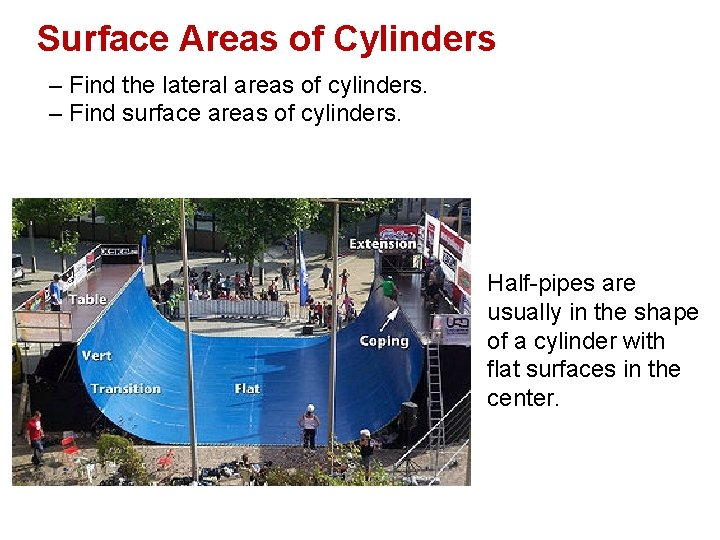Surface Areas of Cylinders – Find the lateral areas of cylinders. – Find surface areas of cylinders. Half-pipes are usually in the shape of a cylinder with flat surfaces in the center.LATERAL AREAS OF CYLINDERS The axis of a cylinder is the segment with endpoints that are centers of its circular bases. Base r Axis If the axis is also the altitude, then the cylinder is called a right cylinder. Otherwise, the cylinder is an oblique cylinder. Altitude Base Right Cylinder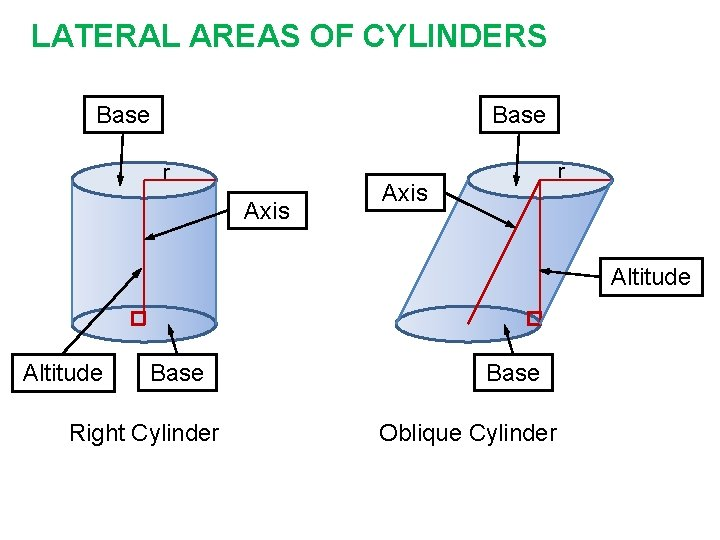LATERAL AREAS OF CYLINDERS Base r Axis Altitude Base Right Cylinder Base Oblique CylinderKey Concept Lateral Area of a Cylinder If a right cylinder has a lateral area of L square units, a height of h units, and the bases have radii of r units, then L = 2 rh. 2 r hExample 1 Lateral Area of a Cylinder MANUFACTURING An office has recycling barrels for cans and paper. The barrels are cylindrical with cardboard sides and plastic lids and bases. Each barrel is 3 feet tall, and the diameter is 30 inches. How many square feet of cardboard are used to make each barrel?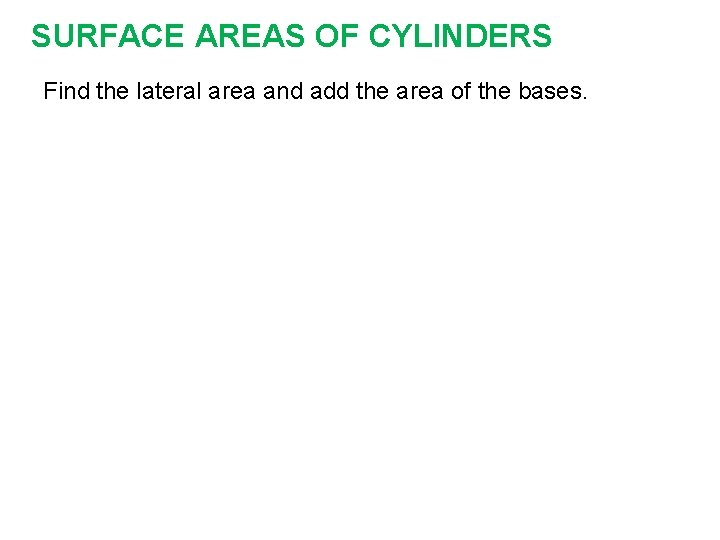SURFACE AREAS OF CYLINDERS Find the lateral area and add the area of the bases.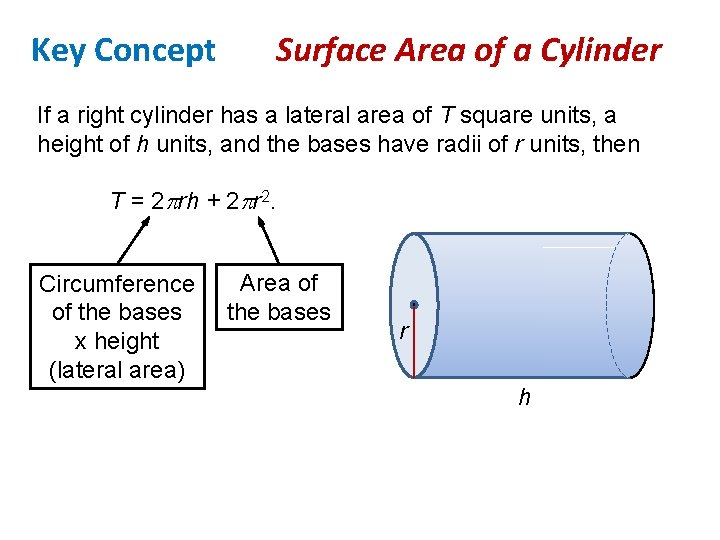Key Concept Surface Area of a Cylinder If a right cylinder has a lateral area of T square units, a height of h units, and the bases have radii of r units, then T = 2 rh + 2 r 2. Circumference of the bases x height (lateral area) Area of the bases r h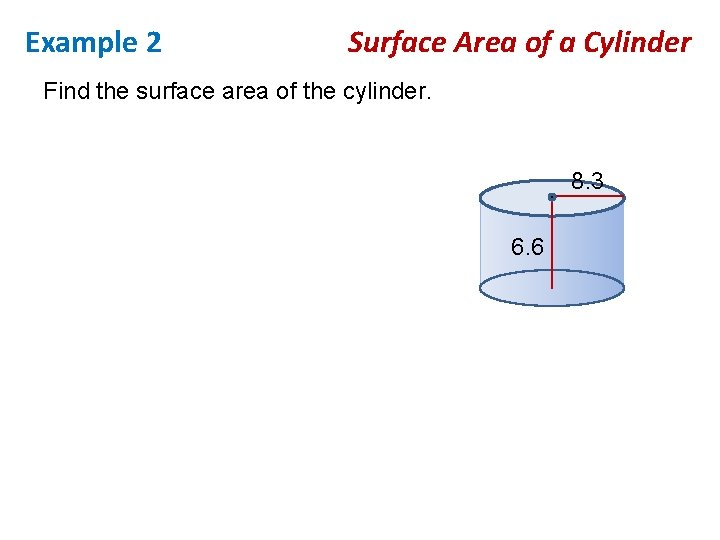Example 2 Surface Area of a Cylinder Find the surface area of the cylinder. 8. 3 6. 6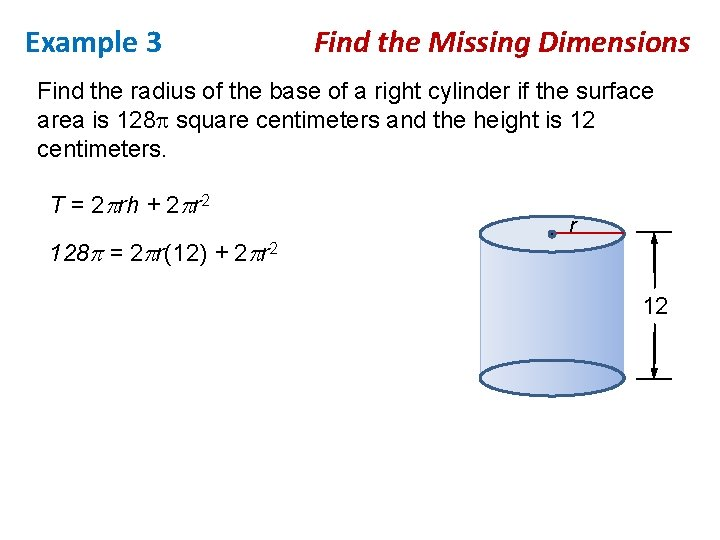Example 3 Find the Missing Dimensions Find the radius of the base of a right cylinder if the surface area is 128 square centimeters and the height is 12 centimeters. T = 2 rh + 2 r 2 128 = 2 r(12) + 2 r 12